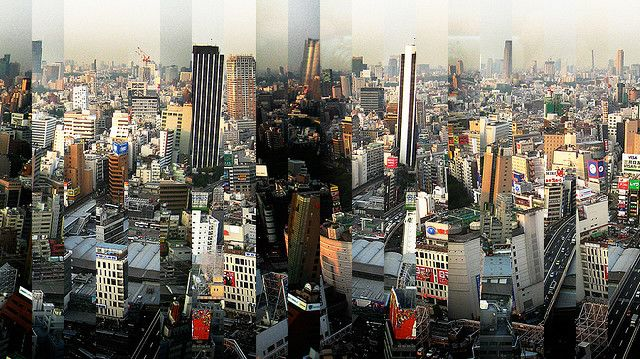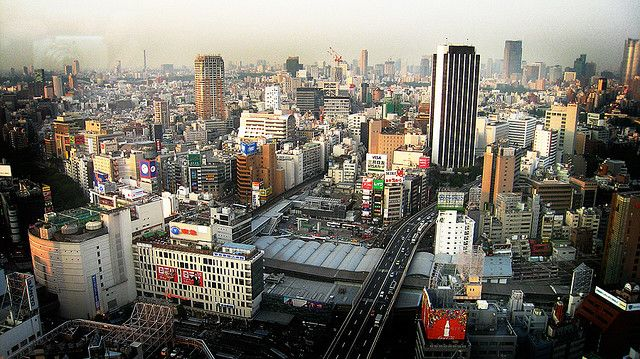Today I have read about the Instagram Engineering Challenge: The Unshredder, and decided to give it a try. The task is simple to explain: Create a program that can unshred this image (do not try the challenge on this image, try the original PNG source instead!):I have postet here that I think I can solve it in 2 hours, and got some downvotes for that; so I have decided to really give it a try. Long story short, it took me about 2 hours and 35 minutes. I’m sure others can do it much faster too.

Before I started developing, I made some quick assumptions to simplify things:

• I want to code it in Ruby, this is the language where I am most productive.
• I can assume the size of the stripes is well known, and I have hardcoded this size.
• The image can be converted to RAW with an external tool, and written into RAW.

While coding I timed myself, and created a little timeline of my trials and errors. Since I wanted to finish as quickly as possible, the code is very ugly: no tests, some hardcoded constants, etc.

## Timeline

I coded this with a stopwatch, and created a timeline of my what has happened every few minute:

• `0:08` - how to read binary files…
• `0:14` - index RGB values
• `0:21` - difference calculation seems to privide a reasonable number
• `0:25` - create all pairings of each row (too slow: need to use logic for all slices)
• `0:30` - create all pairings
• `0:31` - sorted pairings
• `0:35` - copy and number image to mspaint to verify
• `0:36` - bugfix, wrong slice row calculation
• `0:48` - getting stuck with combining pairs
• `1:03` - recombining
• `1:14` - thinking about how to ensure not to combine invalid groups (duplicates!)
• `1:33` - got first reasonable order. Hopefully the correct order!!
• `1:47` - wrote first image, wrong order
• `1:53` - got an almost correct image! one slice was aligned wrong.
• `1:58` - no luck with grayscale
• `2:28` - damn black-white skyscraper!
• `2:29` - SUCCESSSS!!!!!!

Time not measured: I thought about the problem about 5 minutes before starting hacking. So my time is probably about 2:34.

## Code

Without further ado, I give you the code:

``````class Img
attr_reader :w, :h, :slices
def initialize
# unpack as 8bit unsigned chars
@w = 640
@h = 359
@slices = 20
@sw = @w / @slices
end

def write(sorted)
data = @d.dup
source_slice = 0
sorted.each do |target_slice|
# copy one slice
@sw.times do |w|
@h.times do |h|
target_idx = idx(w + @sw * source_slice, h)
source_idx = idx(w + @sw * target_slice, h)
data[target_idx] = @d[source_idx]
data[target_idx+1] = @d[source_idx+1]
data[target_idx+2] = @d[source_idx+2]
end
end
source_slice += 1
end

File.open("out.raw", "wb") do |f|
f.write data.pack("C*")
end
end

def idx(x, y)
(y * @w + x) * 3
end

# top left is 0, 0
def rgb(x, y)
i = idx(x, y)
[@d[i], @d[i+1], @d[i+2]]
end

# calculate sum of difference between r, g, b of two columns
def difference(x1, x2)
diff = 0
@h.times do |y|
# find best in range. This is required because otherwise the black syscraper fucks things up.
lower = y - 15
lower = 0 if (lower < 0)

upper = y + 15
upper = @h-1 if upper >= @h

best = 1e100
lower.upto(upper) do |r|
v = 0
rgb1 = rgb(x1, r)
rgb2 = rgb(x2, r)

d = rgb1 - rgb2
v += d*d
d = rgb1 - rgb2
v += d*d
d = rgb1 - rgb2
v += d*d

best = v if (v < best)
end
diff += best
end
diff
end

def slice_start_idx(s)
@sw * s
end

def slice_end_idx(s)
@sw * s + @sw - 1
end
end

# calculate
img = Img.new

# create ALL pairings.
slices = 20
pairs = []
slices.times do |s1|
slices.times do |s2|
next if s1 == s2

pairs.push [img.difference(img.slice_end_idx(s1), img.slice_start_idx(s2)), [s1, s2] ]
end
end

# create array of fixed numbers
taken_numbers = {}
taken_left = {}
taken_right = {}
combined.each do |group|
group[1..-2].each do |x|
taken_numbers[x] = true
end
taken_left[group.last] = true
taken_right[group.first] = true
end

was_found = false
combined.each do |group|
next if was_found
if (group.last == new_group.first)
# insert at back
was_found = true
new_group.delete_at(0)
if taken_numbers[new_group.last] || taken_left[new_group.last] || new_group.last == group.first
return
end
new_group.each do |x|
group.push x
end
elsif (group.first == new_group.last)
# insert at front
was_found = true
new_group.pop
if taken_numbers[new_group.first] || taken_right[new_group.first] || new_group.first == group.last
return
end
new_group.reverse.each do |x|
group.insert(0, x)
end
end
end
if !was_found
cf = combined.flatten
new_group.each do |x|
was_found = was_found || cf.index(x)
end
if !was_found
combined.push new_group
end
end
end

# sort, lowest first
pairs.sort!

# create combinations.
# combined has an array of all correspondences.
combined = []
pairs.each do |diff, new_group|

# insert new group

# try to recombine everything, until nothing changes any more
was_recombined = true
while was_recombined
new_combined = []
combined.each do |group|
end
was_recombined = combined.size != new_combined.size
combined = new_combined
end

if (combined.size == 1 && combined.size == img.slices)
# we got everything! Write output image.
img.write(combined)
exit
end
end
``````

## Result

Here is the result I got with this code:## Algorithm

In hindsight, the algorithm combines two ideas: Hierarchical Agglomerative Clustering, and a quick and dirty novel distance-metric that defines how “good” a pairing is.

The hierarchical agglomerative clustering is a bit difficult to code, because there are a lot of corner cases when you are allowed to combine pairings and when not. I am not sure if my code is correctly, this should be recoded without time pressure.

The first version of the distance metric was very simple: calculate the sum of the quadratic differences between the two neighboring pixels when two slices are put together. Unfortunately this does not work for the slices with skyscraper that has black-white stripes: here lots of pixels are white on the left side, but black on the right side since the building is just slightly tilt. After realizing this problem, the solution is simple: for each pixel, find the best matching pixel of the other slice within a certain range. Through trial and error I have chosen +-15 pixels, and finally got the correct image.

Happy hacking!

Martin